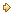Visitors Online: 77 | Friday 24th May 2019

CBSE Guess > Papers > Question Papers > Class XII > 2004 > Economics > Compartment Outside Delhi Set-II

ECONOMICS (Set II—Compartment Outside Delhi)

Except for the following questions, all the remaining questions have been asked in Set I.

SECTION - A

Q. 1. Answer the following questions: 1x4
(i) Give one example of fixed cost.
(ii) Give meaning of producer’s equilibrium.
(iii) Define market period.
(iv) State what economics is all about.

Q. 6. State three features of perfect competition. Draw Average Revenue Curve of a firm in this type of market. 4
Or
State three features of monopolistic competition. Draw Average Revenue Curve of a firm in this market.

Q. 7. Complete the following table: 4

 Output (units) Total Revenue (Rs.) Marginal Revenue (Rs.) Price (Rs.) 1 2 3 4 4 6 6 4 — — — — — — — —

Q. 9. A consumer spends Rs. 90 on a good when its price is Rs. 9 per unit. When the price falls to Rs. 6 per unit, he spends Rs. 120. Calculate the price elasticity by percentage method. 4

SECTION - B

Q. 16. If marginal propensity to consume is 0.2 and increase in investment is Rs. 120 crores, calculate increase in national incomes. 3

Q. 17. Calculate Net Value Added at Market Price from the following: 3

 (Rs. in lkhs) (i) Opening stock (ii) Net indirect tax (iii) Subsidy (iv) Intermediate cost (v) Closing stock (vi) Depreciation (vii) Sales 10 7 2 12 8 5 40

Q. 22. Calculate (a) National Income (b) Net National Disposable Income: 4, 2

 (Rs. in lakhs) (i) Government final consumption expenditure (ii) Net imports (iii) Change in stocks (iv) Consumption of fixed capital (depreciation) (v) Private final consumption expenditure (vi) Net factor income from abroad (vii) Net domestic capital formation (viii) Net current transfers from abroad (ix) Net indirect tax 60 10 5 20 250 (-) 5 40 10 15

 Economics 2004 Question Papers Class XII Delhi Outside Delhi Compartment Delhi Compartment Outside DelhiSet ISet ISet ISet ISet IISet IISet IISet IISet IIISet III

CBSE 2004 Question Papers Class XII